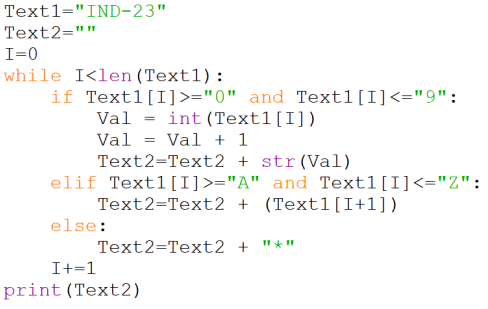CBSE Class 12 Sample Paper for 2024 Boards

Computer Science - Class 12
Solutions to CBSE Sample Paper - Computer Science Class 12

##googletag.cmd.push(function() { googletag.display('div-gpt-ad-1669298377854-0'); });

The output of the Python code is

ND-*34

• The given Python code is a while loop that iterates over each character of the string Text1 and performs some operations based on the character’s value.
• The variable Text2 is initialized as an empty string and is updated with the new characters after each iteration.
• The variable I is used as the index of the current character in Text1 and is incremented by 1 after each iteration.
• The if-elif-else statement inside the loop checks the following conditions for each character:
• If the character is a digit between 0 and 9, then it is converted to an integer using the int() function, and then increased by 1 using the + operator. The result is then converted back to a string using the str() function, and appended to Text2 using the + operator.
• Elif the character is an uppercase letter between A and Z, then Text2 is updated with the next character of Text1 using the [ ] operator for indexing and slicing.
• Else, Text2 is updated with a ‘*’ character.
• The final value of Text2 is printed using the print() function.
• Let us see how the code works for each character of Text1:
• The first character of Text1 is ‘I’, which satisfies the elif condition, since it is between ‘A’ and ‘Z’. Therefore, Text2 is updated with the next character of Text1, which is ‘N’. So, Text2 becomes ‘N’.
• The second character of Text1 is ‘N’, which also satisfies the elif condition, since it is between ‘A’ and ‘Z’. Therefore, Text2 is updated with the next character of Text1, which is ‘D’. So, Text2 becomes ‘ND’.
• The third character of Text1 is ‘-’, which does not satisfy any of the if or elif conditions, since it is neither a digit nor an uppercase letter. Therefore, Text2 is updated with a ‘ ’ character. So, Text2 becomes 'ND- ’.
• The fourth character of Text1 is ‘2’, which satisfies the if condition, since it is a digit between 0 and 9. Therefore, Text2 is updated with the value of 2+1 converted to a string, which is ‘3’. So, Text2 becomes ‘ND-*3’.
• The fifth character of Text1 is ‘3’, which also satisfies the if condition, since it is a digit between 0 and 9. Therefore, Text2 is updated with the value of 3+1 converted to a string, which is ‘4’. So, Text2 becomes ‘ND-*34’.
• The loop ends after the fifth iteration, since there are no more characters in Text1. The final value of Text2 is printed as: ND-*34

So, the correct output of the Python code is:

ND-*34

Learn in your speed, with individual attention - Teachoo Maths 1-on-1 Class